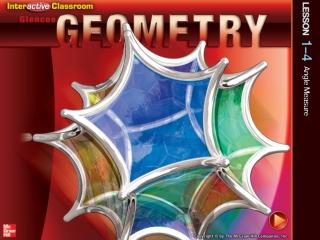DownloadDownload PresentationSplash Screen

# Splash Screen

Télécharger la présentation## Splash Screen

- - - - - - - - - - - - - - - - - - - - - - - - - - - E N D - - - - - - - - - - - - - - - - - - - - - - - - - - -
##### Presentation Transcript

1. Splash Screen

2. Five-Minute Check (over Lesson 1–3) CCSS Then/Now New Vocabulary Example 1: Real-World Example: Angles and Their Parts Key Concept: Classify Angles Example 2: Measure and Classify Angles Example 3: Measure and Classify Angles Lesson Menu

3. Use the number line to find the measure of AC. 5-Minute Check 1

4. Use the number line to find the measure of DE. 5-Minute Check 2

5. Use the number line to find the midpoint of EG give the name of the point. 5-Minute Check 3

6. Find the distance between P(–2, 5) and Q(4, –3). 5-Minute Check 4

7. Find the coordinates of Mif it is the midpoint of RS and the coordinates are S(0, –10) and R (-8, 20). 5-Minute Check 5

8. A boat located at (4, 1) can dock at two locations. Location A is at (–2, 9) and Location B is at (9, –11). Which location is closest? How many units away is the closest dock? 5-Minute Check 6

9. Content Standards G.CO.1 Know precise definitions of angle, circle, perpendicular line, parallel line, and line segment, based on the undefined notions of point, line, distance along a line, and distance around a circular arc. G.CO.12 Make formal geometric constructions with a variety of tools and methods (compass and straightedge, string, reflective devices, paper folding, dynamic geometric software, etc.). Mathematical Practices 5 Use appropriate tools strategically. 6 Attend to precision. CCSS

10. You measured line segments. • Measure and classify angles. • Identify and use congruent angles and the bisector of an angle. Then/Now

11. B A • opposite rays – two rays with the same initial point and go in opposite directions. B A C Vocabulary

12. How to draw: Two rays with the same initial point. Angle – made up of two rays with the same initial point. Named by the three letters going around the angle, with the middle letter always being the vertex. C Written: CAB or BAC B Sides: the rays that make up the angle and A Vertex: the initial point of each ray, the corner. point A Interior: the inside of the angle. Exterior: the outside of the angle.

13. Answer: Angles and Their Parts 1.Name all angles that have B as a vertex. Example 1

14. Answer: Angles and Their Parts 2.Name the sides of5. Example 1

15. 3. Angles and Their Parts Example 1

16. A. B. C. D. 4. Example 1a

17. A. B. C. D.none of these 5. Example 1b

18. A. B. C. D. Which of the following is another name for 3? 6. Example 1c

19. An angle that measures EXACTLY90 degrees. An angle whose measure is less than 90, but greater than 0. An angle whose measure is greater than 90, but less than 180. Right Angle Acute Angle Obtuse Angle Concept

20. Measure and Classify Angles 7. Classify TYVas right, acute, or obtuse. Answer:TYV is a right angle. Example 2

21. Measure and Classify Angles 8. Classify WYT as right, acute, or obtuse. Answer:WYT is an obtuse angle. Example 2

22. Measure and Classify Angles 9. Classify TYUas right, acute, or obtuse. Answer:TYUis an acute angle. Example 2

23. 10.Measure CZD and classify it as right, acute, or obtuse. A. 30°, acute B. 30°, obtuse C. 150°, acute D. 150°, obtuse Example 2a

24. 11.Measure CZE and classify it as right, acute, or obtuse. A. 60°, acute B. 90°, acute C. 90°, right D. 90°, obtuse Example 2b

25. 12.Measure DZX and classify it as right, acute, or obtuse. A. 30°, acute B. 30°, obtuse C. 150°, acute D. 150°, obtuse Example 2c

26. Measure and Classify Angles 13. Wall stickers of standard shapes are often used to provide a stimulating environment for a young child’s room. A five-pointed star sticker is shown with vertices labeled. Find mGBH and mHCI if GBH  HCI, mGBH = 2x + 5, and mHCI=3x – 10. Example 3

27. Measure and Classify Angles Step 1 Solve for x. GBH  HCI Given mGBH = mHCI Definition of congruent angles 2x + 5 = 3x – 10 Substitution 2x + 15 = 3x Add 10 to each side. 15 = x Subtract 2x from each side. Example 3

28. . Measure and Classify Angles Step 2 Use the value of x to find the measure of either angle. Answer:mGBH = 35, mHCI = 35 Example 3

29. 14. Find mBHC and mDJE if BHC  DJE, mBHC = 4x + 5, and mDJE = 3x + 30. Example 3

30. degree • right angle • acute angle • obtuse angle • angle bisector • ray • opposite rays • angle • side • vertex • interior • exterior Vocabulary

31. End of the Lesson